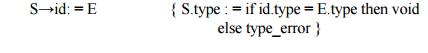Home | | Compiler Design | Specification of a Simple Type Checker

# Specification of a Simple Type Checker

A type checker for a simple language checks the type of each identifier. The type checker is a translation scheme that synthesizes the type of each expression from the types of its subexpressions. The type checker can handle arrays, pointers, statements and functions.

SPECIFICATION OF A SIMPLE TYPE CHECKER

A type checker for a simple language checks the type of each identifier. The type checker is a translation scheme that synthesizes the type of each expression from the types of its subexpressions. The type checker can handle arrays, pointers, statements and functions.

A Simple Language

Consider the following grammar:

P → D ; E

D → D ; D | id : T

T → char | integer | array [ num ] of T | ↑ T

E → literal | num | id | E mod E | E [ E ] | E ↑

Translation scheme:

P → D ; E

D → D ; D

D → id : T { addtype (id.entry , T.type) }

T → char { T.type : = char }

T → integer { T.type : = integer }

T → ↑ T1 { T.type : = pointer(T1.type) }

T → array [ num ] of T1 { T.type : = array ( 1… num.val , T1.type) }

In the above language,

There are two basic types : char and integer ; → type_error is used to signal errors;

the prefix operator ↑ builds a pointer type. Example , ↑ integer leads to the type expression

pointer ( integer ).

Type checking of expressions

In the following rules, the attribute type for E gives the type expression assigned to the expression generated by E.

1. E → literal { E.type : = char } E→num { E.type : = integer }

Here, constants represented by the tokens literal and num have type char and integer.

2. E → id { E.type : = lookup ( id.entry ) }

lookup ( e ) is used to fetch the type saved in the symbol table entry pointed to by e.

3. E → E1 mod E2 { E.type : = if E1. type = integer and E2. type = integer then integer

else type_error }

The expression formed by applying the mod operator to two subexpressions of type integer has type integer; otherwise, its type is type_error.

4. E → E1 [ E2 ] { E.type : = if E2.type = integer and E1.type = array(s,t) then t

else type_error }

In an array reference E1 [ E2 ] , the index expression E2 must have type integer. The result is the element type t obtained from the type array(s,t) of E1.

5. E → E1 ↑ { E.type : = if E1.type = pointer (t) then t

else type_error }

The postfix operator ↑ yields the object pointed to by its operand. The type of E ↑ is the type t of the object pointed to by the pointer E.

Type checking of statements

Statements do not have values; hence the basic type void can be assigned to them. If an error is detected within a statement, then type_error is assigned.

Translation scheme for checking the type of statements:

1.     Assignment statement: S→id: = E2. Conditional statement: S→if E then S13. While statement:

S → while E do S14. Sequence of statements:

S → S1 ; S2 { S.type : = if S1.type = void and S1.type = void then void else type_error }Type checking of functions

The rule for checking the type of a function application is : E → E1 ( E2) { E.type : = if E2.type = s and

E1.type = s → t then t else type_error }

Study Material, Lecturing Notes, Assignment, Reference, Wiki description explanation, brief detail
Principles of Compiler Design : Syntax Analysis and Run-Time Environments : Specification of a Simple Type Checker |